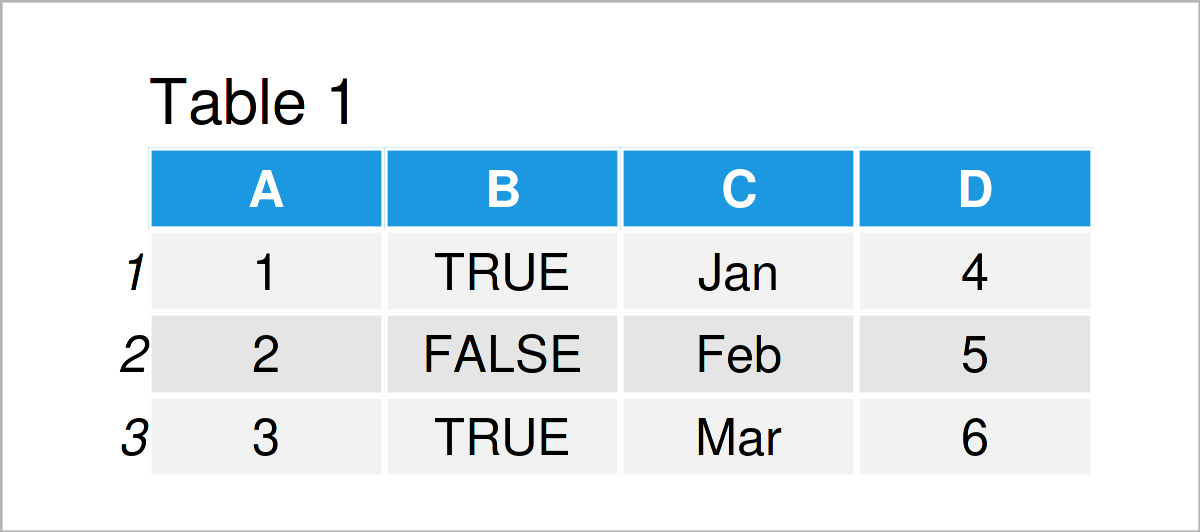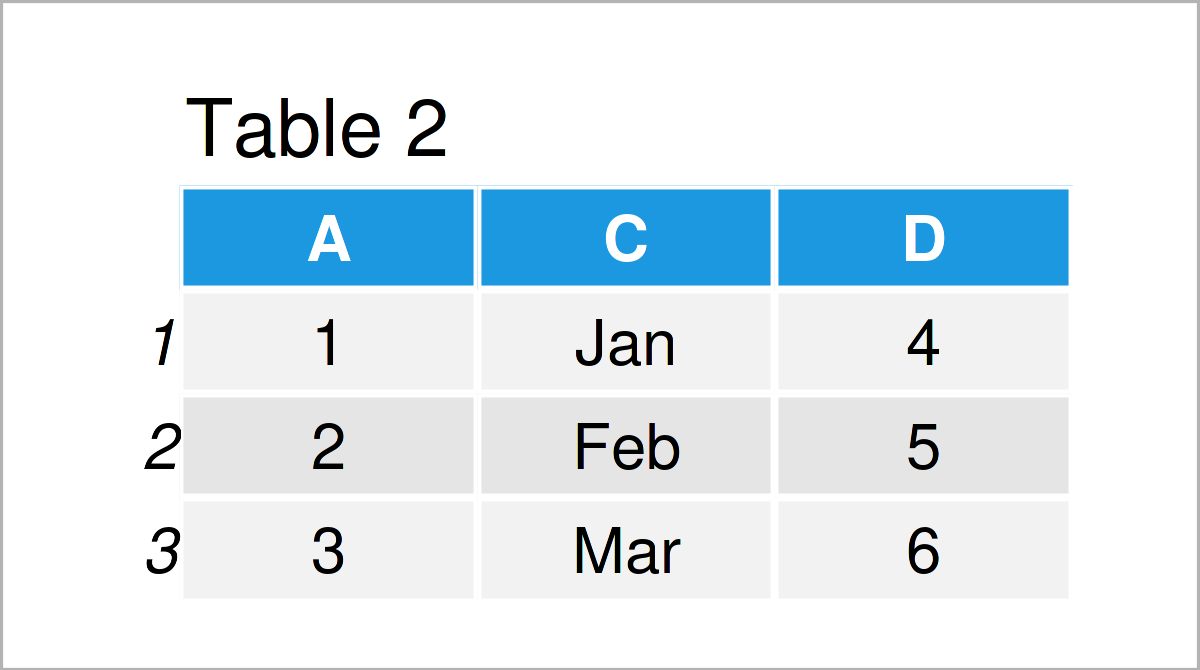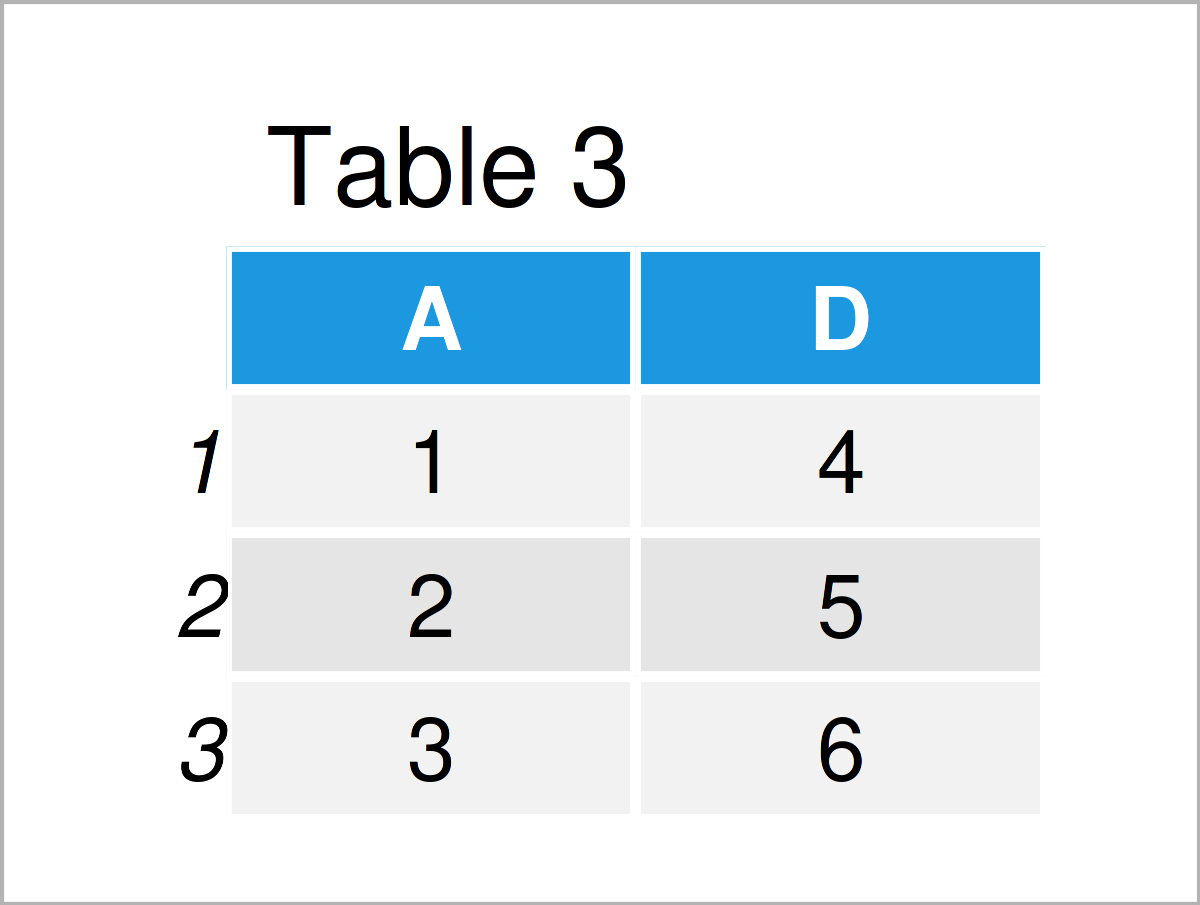# Delete Column of data.table by Index in R (2 Examples)

In this tutorial, you’ll learn how to remove a column from a data.table by its index in R.

The tutorial contains two examples for the deletion of data.table columns by index. To be more precise, the page will consist of this content:

Let’s dive right in.

## Example Data & Packages

If we want to use the functions of the data.table package, we first have to install and load data.table:

```install.packages("data.table")    # Install data.table package

I’ll use the following data as a basement for this R programming tutorial.

```DT_1 <- data.table(A = 1:3,
B = c(TRUE, FALSE, TRUE),
C = month.abb[1:3],
D = 4:6)Table 1 visualizes the output of the RStudio console and shows that our example data consists of four columns.

## Example 1: Delete One Column By Index

In this example, I’ll illustrate how to delete a single column by its index. We delete column number two.

```DT_2 <- data.table::copy(DT_1)    # Replicate data set DT_1
DT_3 <- DT_2[ , -c(2) ]
DT_3```In Table 2 you can see the resulting data.table. The column with column name B was deleted.

## Example 2: Delete Multiple Columns By Index

In Example 2, I’ll illustrate how to remove multiple columns by their indices. We remove columns number two and three.

```DT_4 <- data.table::copy(DT_1)    # Replicate data set DT_1
DT_5 <- DT_4[ , -c(2, 3) ]
DT_5```As shown in Table 3, the previous R syntax has created a data.table, consisting only of the remaining columns with names A and D.

## Video & Further Resources

Would you like to know more about the deletion of data.table columns by index? Then you may have a look at the following video on my YouTube channel. In the video tutorial, I’m explaining the R programming codes of this tutorial.

In addition, you might want to have a look at the other articles on statisticsglobe.com. A selection of interesting articles that are related to the deletion of data.table columns by index is shown below.

In this R programming article, you have learned how to cut out and drop columns of a data.table by index. If you have further questions, please let me know in the comments section below.

This page was created in collaboration with Anna-Lena Wölwer. Have a look at Anna-Lena’s author page to get further information about her academic background and the other articles she has written for Statistics Globe.

Subscribe to the Statistics Globe Newsletter